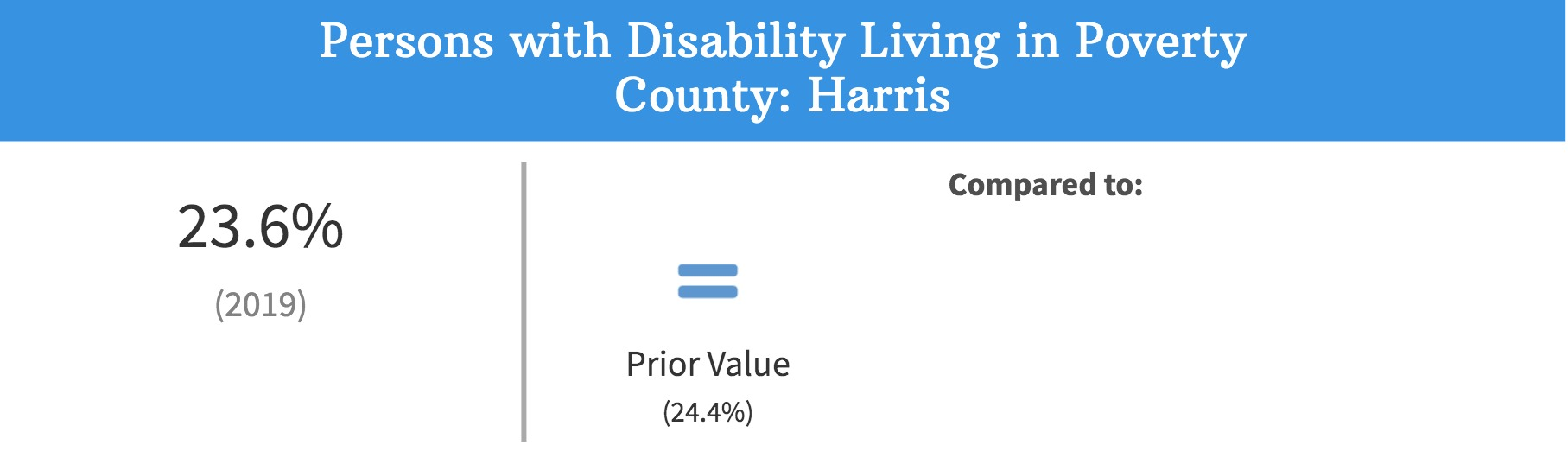# Why is there an equal sign icon when the prior value is different?

The comparison to a prior value shows an equal icon when the confidence intervals overlap. Not all indicators have confidence intervals, but when they do, they are taken into consideration in determining the trend from the previous measurement period.One type of comparison that you'll notice throughout the site is the compare to a prior value.  This comparison displays with green or red arrows depending on whether the value is improving or getting worse or with an equal sign if there is no significant change.

When conducting a survey, researchers only sample a small portion of the entire population who they believe is representative of everyone.  Researchers use two important statistics to measure how well the survey represents everyone - the margin of error and the confidence level.

For example, a survey was conducted that measured the obesity rate as 35% with a 95% confidence level and a margin of error of 5%. We would then know that 95 out of 100 times the survey was conducted, the measured percent of the population that is obese would be between 30% and 40%.  In this case the confidence level is 95%, the margin of error is 5% and the confidence interval is 30% - 40%.

If we have the confidence intervals when calculating a change over time, we take them into account when determining if there was a statistically significant change. If we use the same example above, but the next year the survey measures the obesity rate as 38% with a confidence interval of 35% - 41%, the confidence intervals overlap meaning there is no significant change. In other words, since in the first year the value could be between 30% - 40% and in the second year it could be between 35% and 41%, we don't actually know if there was a change in the rate.